Get instant live expert help with Excel or Google Sheets“My Excelchat expert helped me in less than 20 minutes, saving me what would have been 5 hours of work!”

#### Post your problem and you’ll get expert help in seconds.

Your message must be at least 40 characters
Our professional experts are available now. Your privacy is guaranteed.

# Tips on How to Sum Time in Excel

Time value is stored as a serial number in Excel and sometimes it is tricky to get the accurate result while adding time values in Excel. We need to make sure to use the right approach while making calculations to get an accurate sum of time values. In this article, we will learn how to sum time in Excel without an error or wrong calculation.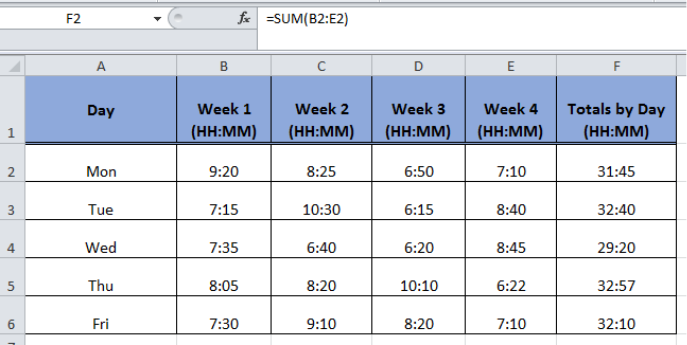Figure 1. The Output of Custom Format Type

## Using SUM Formula

Suppose we want to estimate the total time in hours and minutes while adding up the time values. We have time values for each day of the weeks in terms of hours and minutes worked during two weeks and we can easily calculate the sum of time values by entering the SUM formula in an active cell in Excel, such as;

`=SUM(B2:C2)`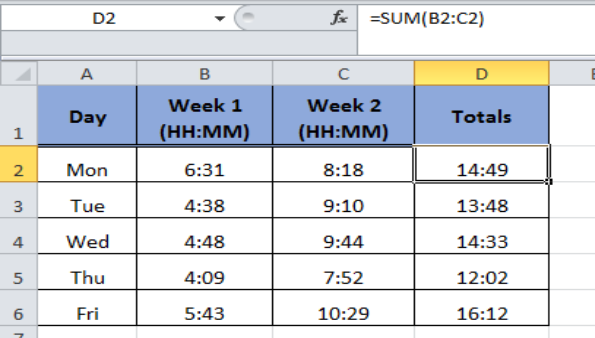Figure 2. Using the SUM Formula

## Using The AutoSum Function

Instead of inserting the SUM formula, we can also use the AutoSum function to add up the time values in Excel. In the above example and using the AutoSum function we can learn how to sum time in Excel in the following steps:

• Select the active cell or cells where we need to sum the time, say D2:D6 in this example,
• Then on the Home tab, in the Editing section choose AutoSum.
• Press Enter to get the result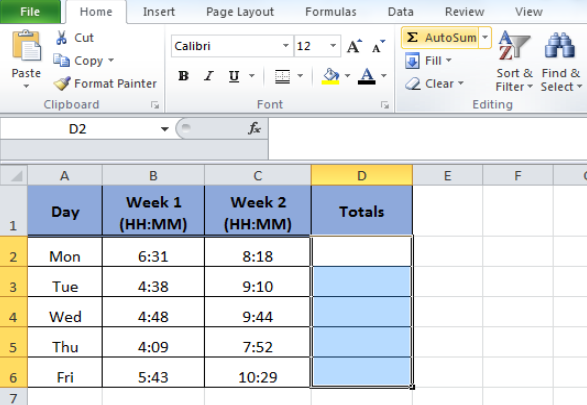Figure 3. Using The AutoSum Function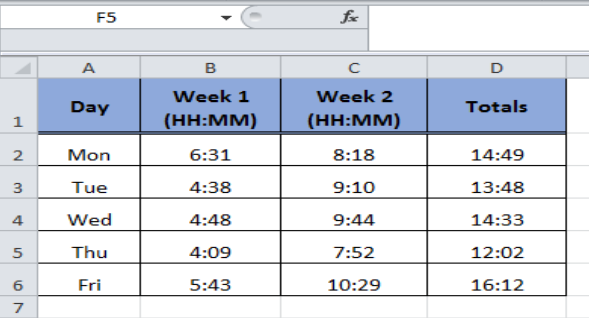Figure 4. The Output of the AutoSum Function

## To Add Up More Than 24 Hours

While making the calculation of time values it happens that the hours add up to more than 24 hours and if we do not select the right format of time the SUM formula or AutoSum function displays the remainder of time instead of the actual sum of time values.

Let’s have a look at the total of time values of all the four weeks in column F. The sum of time values is not what it should be. Because of the sum of time values exceeds 24 hours, so the SUM formula returns the remainder of time and not the actual total of time.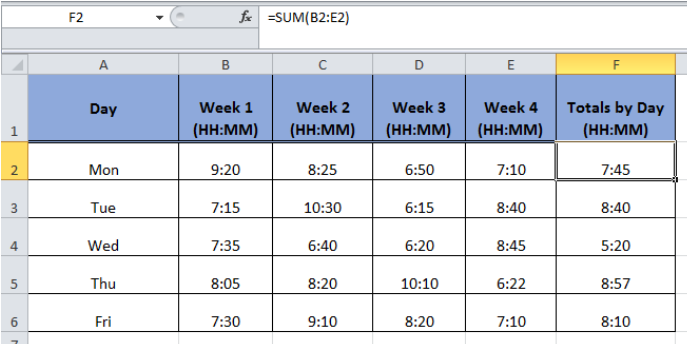Figure 5. The Output of the SUM of Time Values Exceeding 24 hours

In order to get the accurate result, we need to choose the right format type of time that will show the accurate values while performing how to sum time in Excel in the following steps;

• Select the cell or cells to show the sum as more than 24 hours
• Mouse right click and go to Format Cells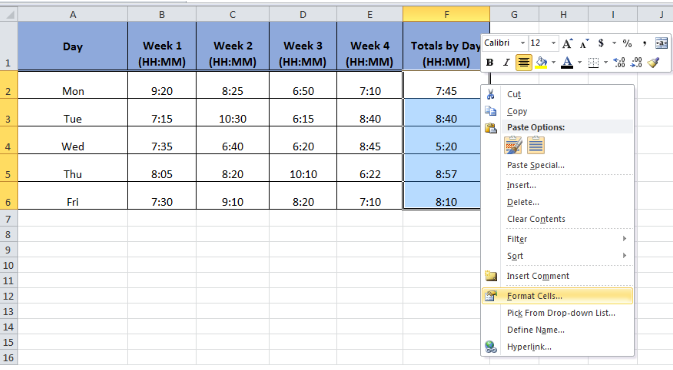Figure 6. Applying the Time Format

• Choose Custom in the Category list.
• In the Type box, at the top of the list of formats, type [h]:mm;@ and press OK.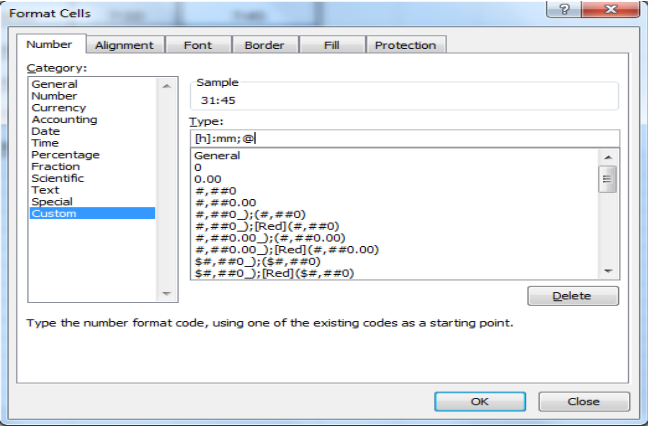Figure 7. Applying the Custom Format Type

This format type will show us the accurate result of Total of the time values in Excel and will be available in the Type list for future use.Figure 8. The Output of Custom Format Type

## Instant Connection to an Expert through our Excelchat Service

Most of the time, the problem you will need to solve will be more complex than a simple application of a formula or function. If you want to save hours of research and frustration, try our live Excelchat service! Our Excel Experts are available 24/7 to answer any Excel question you may have. We guarantee a connection within 30 seconds and a customized solution within 20 minutes.

Solution examplesI need a formula to pop at the upper left corner of a spreadsheet. If I enter the month "January," I want the column number sum of January =SUM(AB11:AB75) from another section on the same excel page to pop right below the "January" cell, and not display the formula expression, but see the \$100.
Solved by T. Q. in 40 minsCan't add (SUM) in imported numbers from bank account
Solved by F. C. in 40 minsI need a formula to combine D2 to D100 to add together a column of numbers, then take away the same amount on the same row when column E is filled. i.e. column D is a price of an item, so the formula must calculate the total, then when the item is sold an 'a' is marked next to the item in column E, the formula then must deduct this amount from the total
Solved by X. W. in 20 minsI would like to have a diagram in a new sheet, where the horizontal axis is the days, as they are in column DX. Each day shall show the sum of all unique leads of that day, and I would like to be able to check via a box of checkboxes, which facilities are shown, the facilities are in column BC.
Solved by I. A. in 45 minsI am working on a cash flow projection. Part of the projection includes sales commissions. Our sales guys earn a monthly draw and then commission on sales after a certain amount. For example they may earn a monthly salary of 12,500 and earn additional commission after their commission equals \$150,000. What I need excel to do is sum a column if the values in the preceeding columns are greater than \$150,000. I've tried using the sumif and the if function in excel and it's not working correctly either way. Any suggestions?
Solved by G. W. in 19 mins